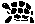# Metacza: Examples

## Fibonacci, Variant 1

```#! metacza --style=metacza

#include <metacza.h>

fib(n) = fib(n-1) + fib(n-2)
fib(0) = 0
fib(1) = 1

```

## Fibonacci, Variant 2

```#! metacza --style=metacza

#include <metacza.h>

fib(n) =
0 if n == 0 else
1 if n == 1 else
(fib(n-1) + fib(n-2))

```

## Sum of List

```#! metacza --style=boost

#include <boost/mpl/int.hpp>
#include <boost/mpl/plus.hpp>

sum(...)
sum() = 0
sum(h,t...) = h + sum(t...)

```

## Lambda 1

```#! metacza --style=metacza

#include <metacza.h>

data list(...)

cons(_,_)
cons(x,list(y...)) = list(x,y...)

map(_, ...)
map(f) = list()
map(f, car, cdr...) = cons(f(car), map(f,cdr...))

isOdd = { (x) = (x % 2) != 0 }

_ = print(map(isOdd, 1,2,3,4,5,6,7,8,9))

```

## Tag Dispatch 1, Boost Style

```#! metacza --style=boost

#include <boost/mpl/string.hpp>
#include <boost/mpl/insert_range.hpp>
#include <boost/mpl/plus.hpp>
#include <boost/mpl/print.hpp>

namespace boost::mpl {
// Better syntax needed!  The following extends the 'plus' implementation
// so that strings can be added (to append them).

const string_tag  // declared somewhere in Boost

let
apply(x,y) = insert_range(x,end(x),y)
in
plus_impl(string_tag,string_tag) = raw(void)
}

helloWorld = "Hello" + " " + "World!"

```

This code is not really intuitive. The result of the function plus_impl is not used at all. Instead of raw(void), anything could have been returned. Even a data declaration could have been used (simply because data are functions that return themselves). The local definition of apply is what implements the tag dispatch function. A very weird way of implementing this.

Nicer syntax will be introduced so this can be written in an understandable way. Note that in version 2, Metacza lacks support for invoking such tag dispatched functions: you cannot access the embedded apply function from Metacza. We'll need syntax for that, too.

## Tag Dispatch 2, Metacza Style

```#! metacza --style=metacza

#include <metacza.h>

data string_tag

tag_of(_)

let
var x: raw(char)...;
in
tag_of(::metacza::string(x...)) = string_tag

append_impl(_,_)
append_impl(string_tag,string_tag) = { (x,y) = x + y }

append(x,y) = append_impl(tag_of(x), tag_of(y))(x,y)

helloWorld = append("Hello  ", "World!")

```

This code is not compatible with Boost, but uses only Metacza basics to implement tag dispatch. It works much the same as the Boost thingy, but the generated C++ is just not compatible due to an additional ::type needed in C++ translation that Boost does not use.

## Tag Dispatch 3, Definition of Tags

Boost.MPL adds tags to data types to make tag dispatch possible. This can be done easily in Metacza by using a let declaration.

```#! metacza --style=boost

#include <boost/mpl/int.hpp>

data mydata_tag;

let
tag = mydata_tag
in
data mydata(_,_,_,_)

```

(Ranks as used for Boost.MPL numbers cannot currently defined in Metacza unless raw C++ code is used.)

## 99 Bottles Of Beer

```#! metacza --style=metacza

#include <stdio.h>
#include <metacza.h>

digit(n)
digit(0) = "0"; digit(1) = "1"; digit(2) = "2"; digit(3) = "3"; digit(4) = "4"
digit(5) = "5"; digit(6) = "6"; digit(7) = "7"; digit(8) = "8"; digit(9) = "9"

str(n) = str(n/10) + digit(n%10)
str(0) = ""

bottles(n) = str(n) + " bottles"
bottles(1) = "1 bottle"
bottles(0) = "No more bottles"

verse(n) = bottles(n) + " of beer on the wall,\n" +
bottles(n) + " of beer.\n" +
"Take one down and pass it around,\n" +
bottles(n-1) + " of beer on the wall.\n"

verse(0) = "No more bottles of beer on the wall,\n" +
"No more bottles of beer.\n" +
"Go to the store and buy some more,\n" +
"99 bottles of beer on the wall.\n"

song(n) = verse(n) + "\n" + song(n-1)
song(0) = verse(0)

beerSong = song(99)

%{
int main(void)
{
puts(metacza::evaluate<beerSong>::value);
return 0;
}
%}

```

To actually see the text, this must be linked and run. The C++ code simply prints a string constant precomputed by the compiler.

## Index

December 4th, 2011
Comments? Suggestions? Corrections? You can drop me a line.
zpentrabvagiktu@theiling.deDatenschutz